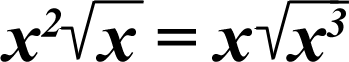# Square Root Squabble

## A real number squared multiplied by its square root is equal to the number multiplied by the square root of its cube!## Examples:

42 × 4^½ = 16 × 2 = 32

4 × (43)^½ = 4 × 64^½ = 4 × 8 = 32

92 × 9^½ = 81 × 3 = 243

9 × (93)^½ = 9 × 729^½ = 9 × 27 = 243

252 × 25^½ = 625 × 5 = 3,125

25 × (253)^½ = 25 × 15,625^½ = 25 × 125 = 3,125

The caret (^) is the symbol of exponentiation. Raising a number to the power of ½ gives you the square root.